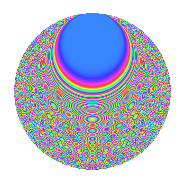# Properties

 Label 2365.2.mLevel 2365 Weight 2 Character orbit m Rep. character $$\chi_{2365}(1332,\cdot)$$ Character field $$\Q(\zeta_{4})$$ Dimension 440 Sturm bound 528

# Related objects

## Defining parameters

 Level: $$N$$ = $$2365 = 5 \cdot 11 \cdot 43$$ Weight: $$k$$ = $$2$$ Character orbit: $$[\chi]$$ = 2365.m (of order $$4$$ and degree $$2$$) Character conductor: $$\operatorname{cond}(\chi)$$ = $$215$$ Character field: $$\Q(i)$$ Sturm bound: $$528$$

## Dimensions

The following table gives the dimensions of various subspaces of $$M_{2}(2365, [\chi])$$.

Total New Old
Modular forms 536 440 96
Cusp forms 520 440 80
Eisenstein series 16 0 16

## Trace form

 $$440q + 16q^{6} + O(q^{10})$$ $$440q + 16q^{6} - 456q^{16} - 32q^{21} + 16q^{23} + 48q^{31} - 48q^{35} + 488q^{36} - 88q^{38} - 80q^{40} - 32q^{41} - 8q^{43} - 40q^{47} - 8q^{52} - 16q^{53} + 40q^{57} - 104q^{68} + 168q^{78} - 632q^{81} + 56q^{83} + 72q^{86} + 112q^{87} - 8q^{90} - 176q^{92} - 104q^{95} - 256q^{96} + O(q^{100})$$

## Decomposition of $$S_{2}^{\mathrm{new}}(2365, [\chi])$$ into newform subspaces

The newforms in this space have not yet been added to the LMFDB.

## Decomposition of $$S_{2}^{\mathrm{old}}(2365, [\chi])$$ into lower level spaces

$$S_{2}^{\mathrm{old}}(2365, [\chi]) \cong$$ $$S_{2}^{\mathrm{new}}(215, [\chi])$$$$^{\oplus 2}$$

## Hecke Characteristic Polynomials

There are no characteristic polynomials of Hecke operators in the database